# Telangana Board Question Paper for Class 10th General Science Part 1 2015 In PDF

## Telangana 10th Standard General Science Part 1 Exam Question Paper 2015 with Solutions – Free Download

Telangana Board Class 10 General Science Part 1 2015 Question Paper Solutions are considered an apt study material. It sets the basis for the important questions that are likely to be repeated in the General Science Part 1 Physical Sciences exam. Students can download the PDFs of these solved or unsolved question papers, as per preference. They can click on the links mentioned in the article and download these papers. These interactive PDF links provide students with the printable format of the question papers. The questions and answers are also published on the web-page.

Students are advised to get acquainted with the complex concepts from Class 10 Physical Sciences, as it will help them to crack the exams easily. These solutions also help the students to know the question types that are usually asked in the TS Board SSC Class 10 General Science Part I exam. Students are also urged to practise solving the previous papers of Telangana Board Class 10 for the exams. Doing so will help them to self analyse their performance and their preparation level for the board exams. By solving these paper solutions regularly, students can get an overview of the question paper design and the marks’ distribution in each section. These question paper solutions also help them to scale the difficulty level of the exams.

### Telangana Board Class 10 General Science Part I 2015 Question Paper with Solutions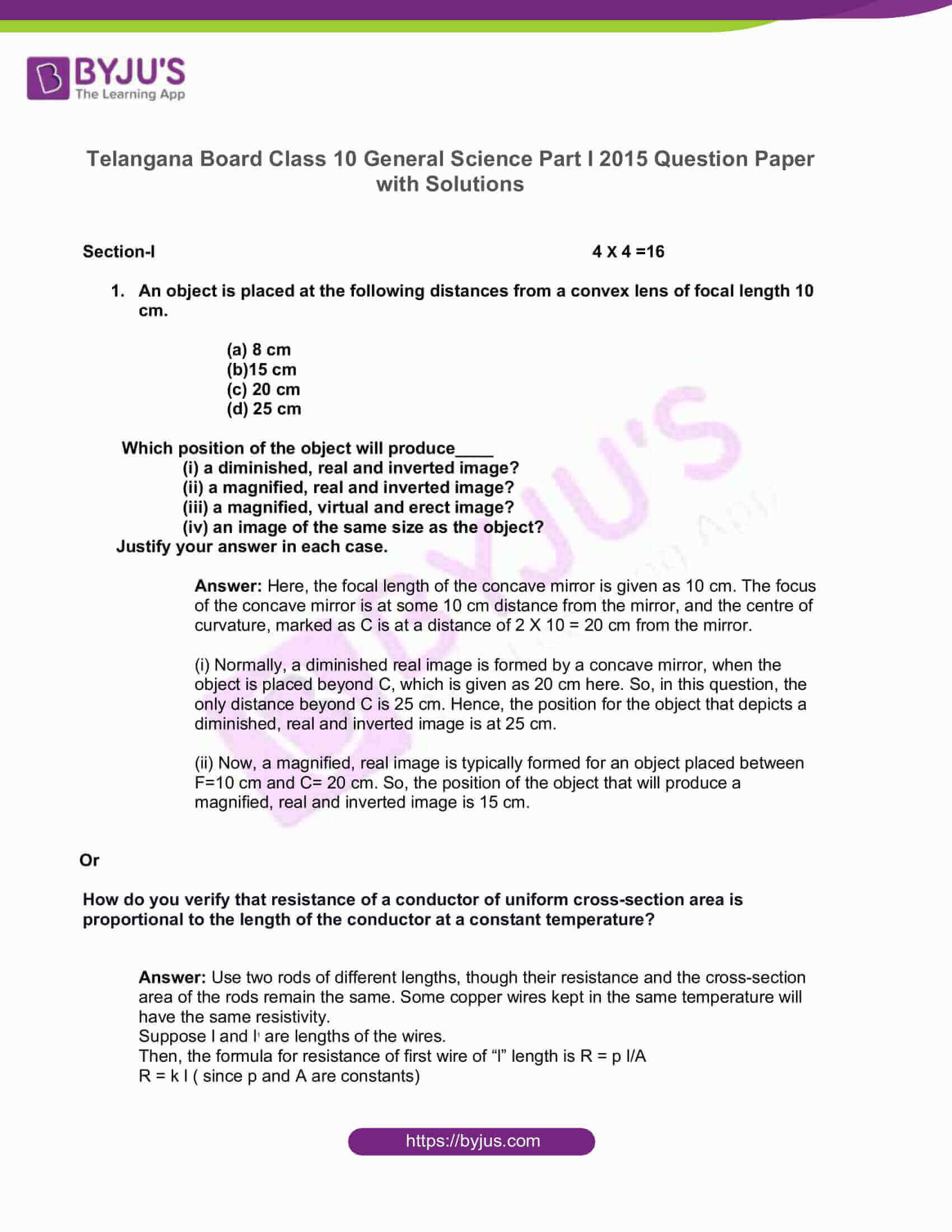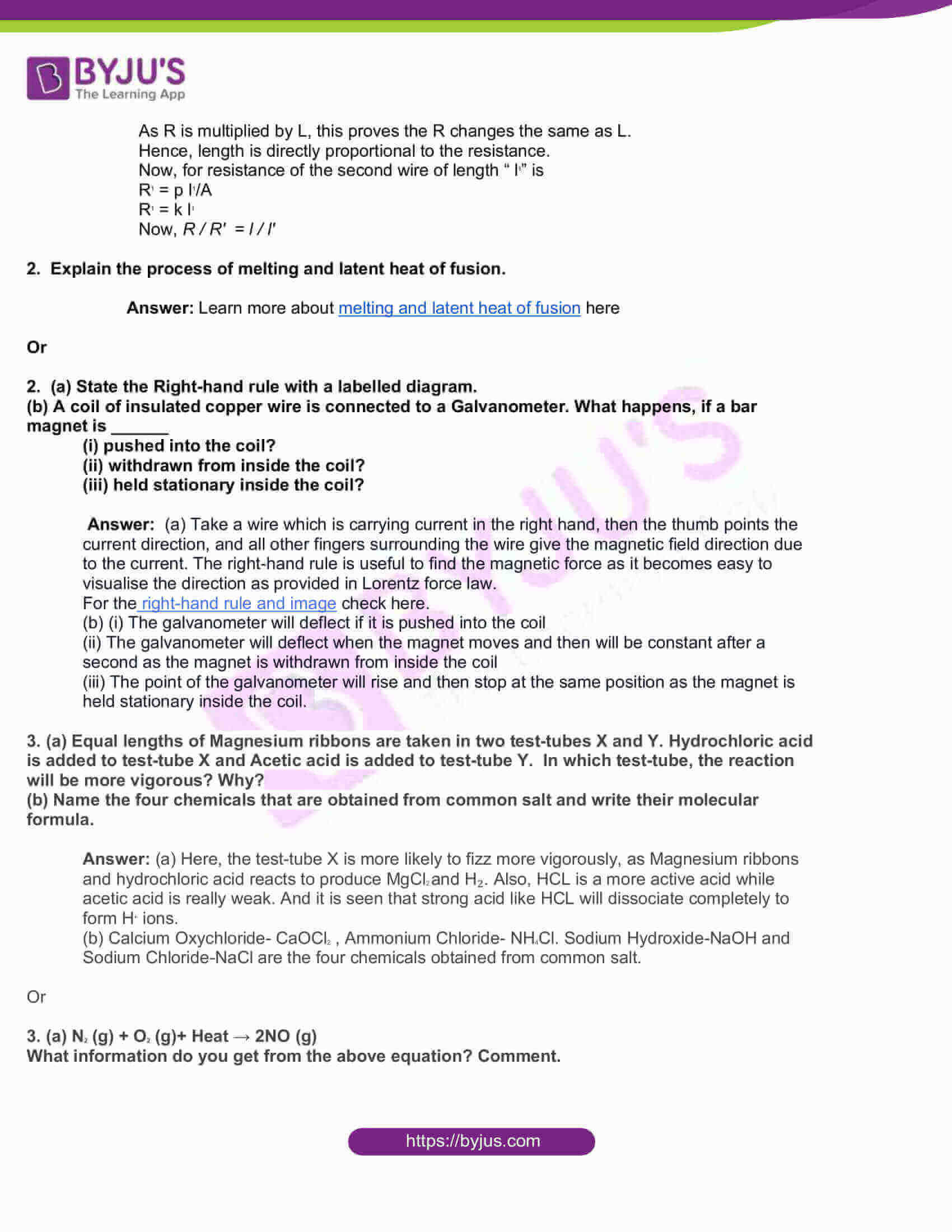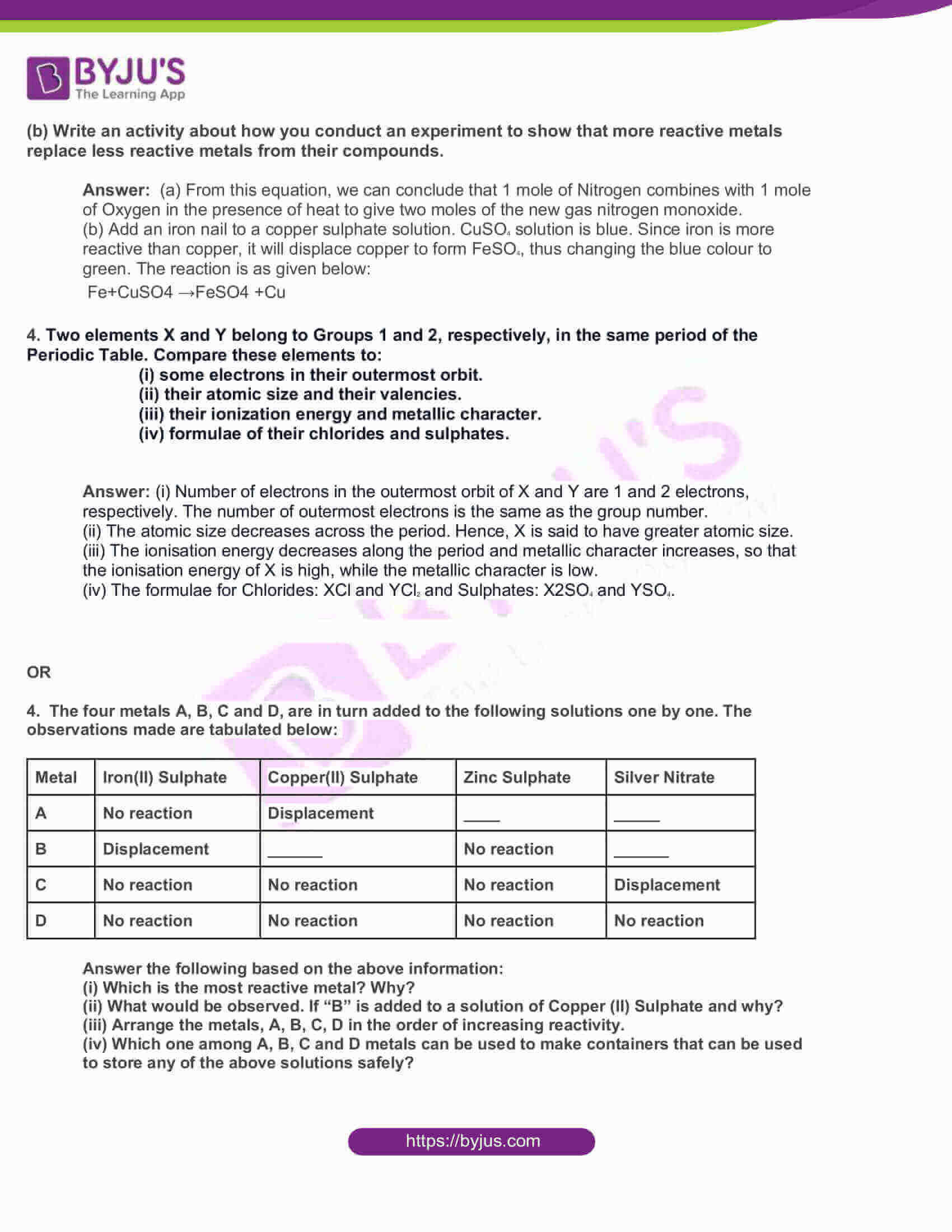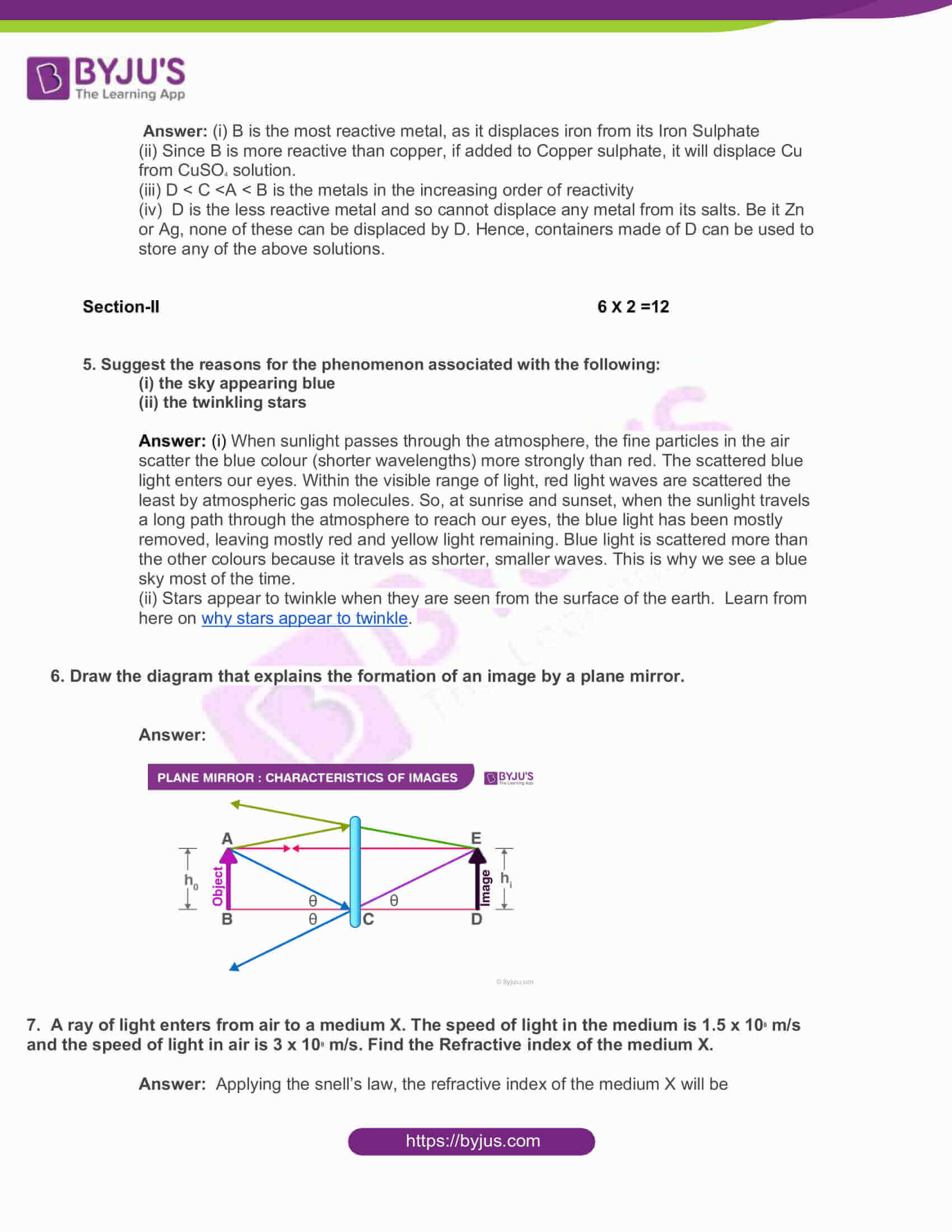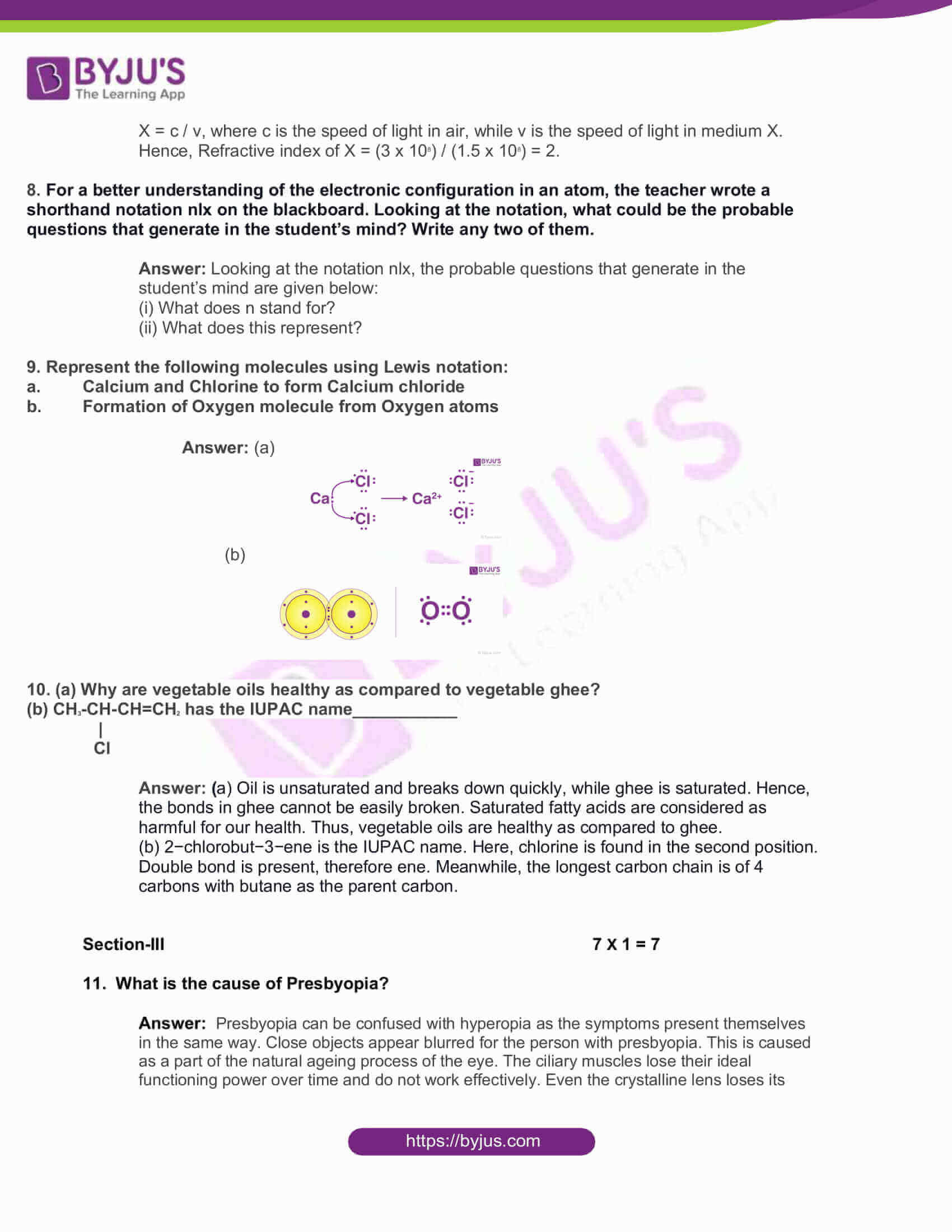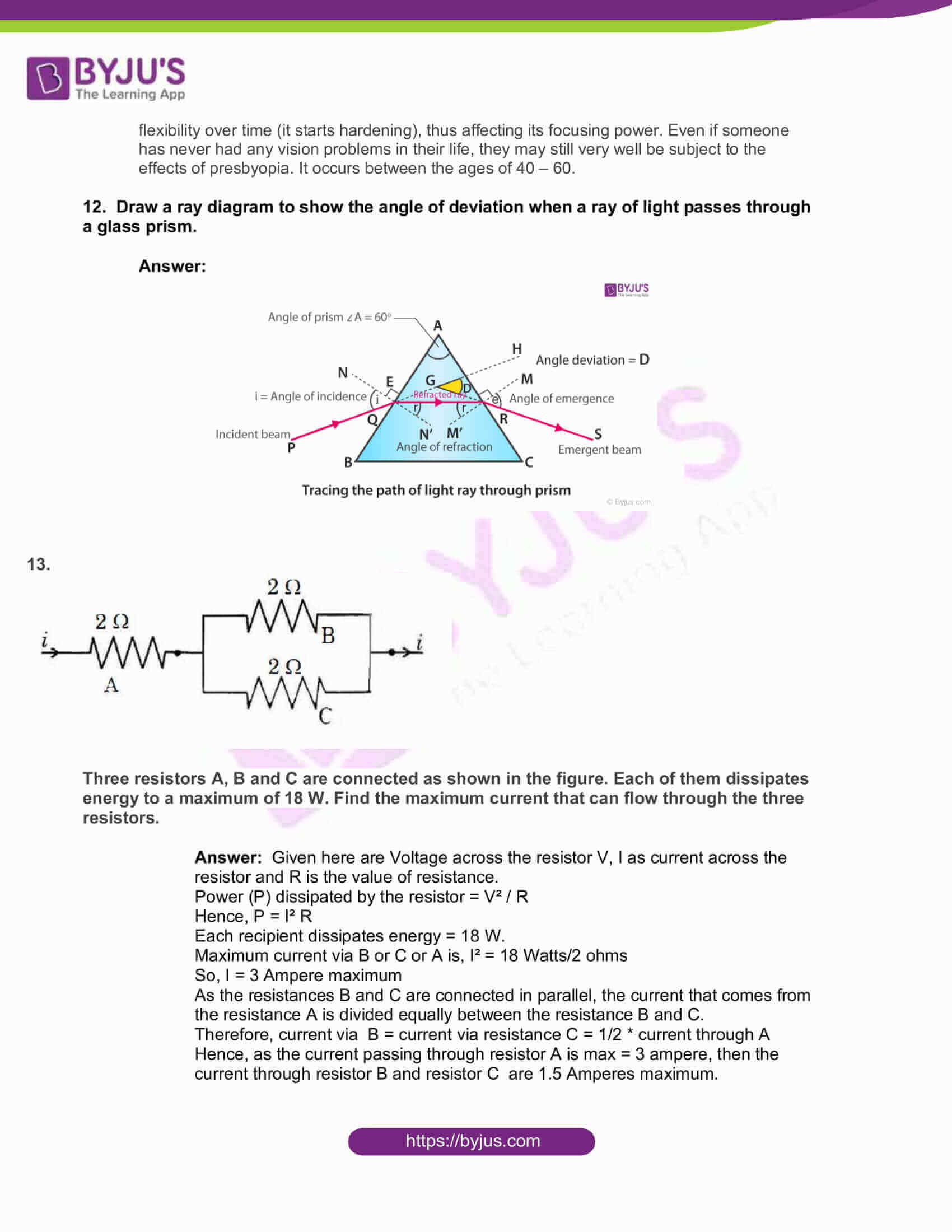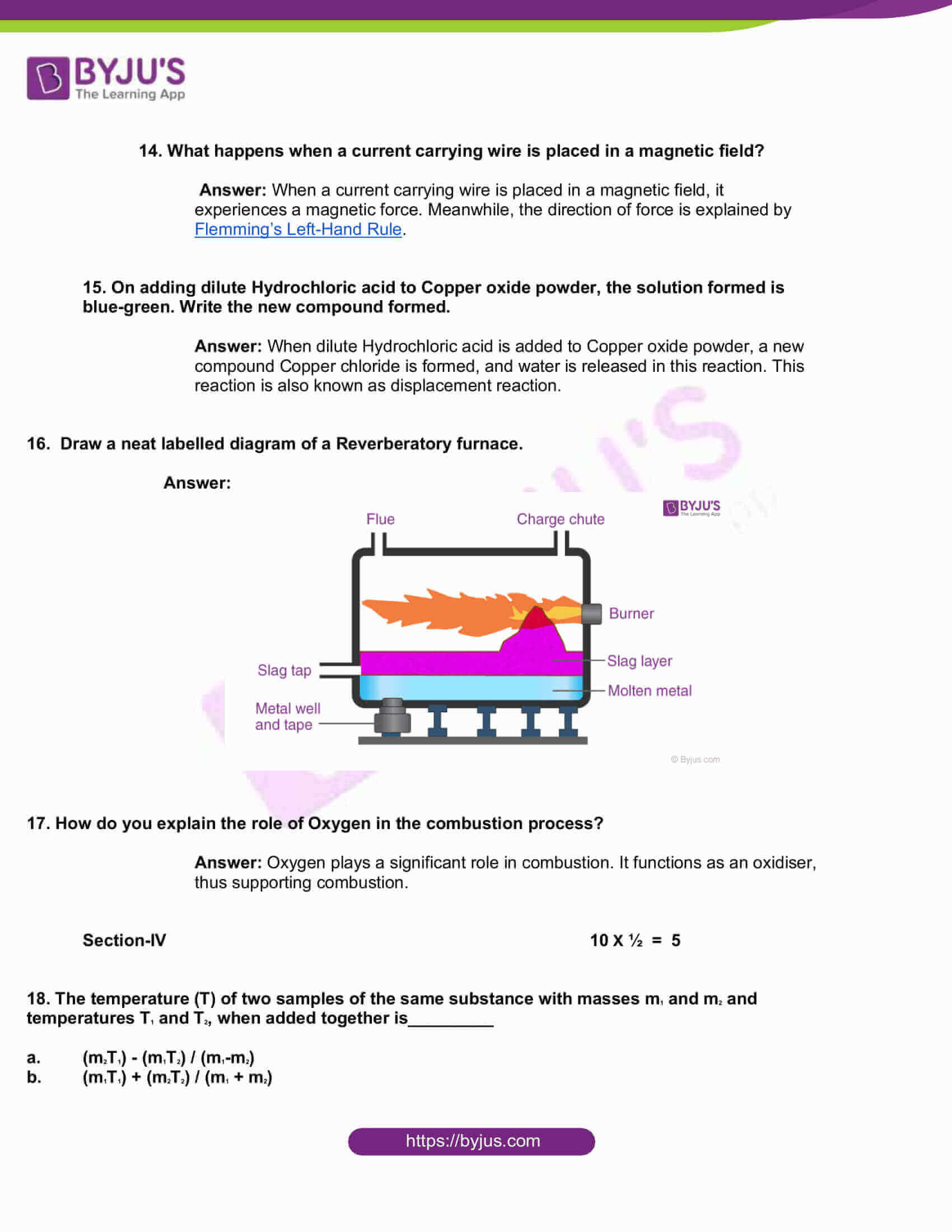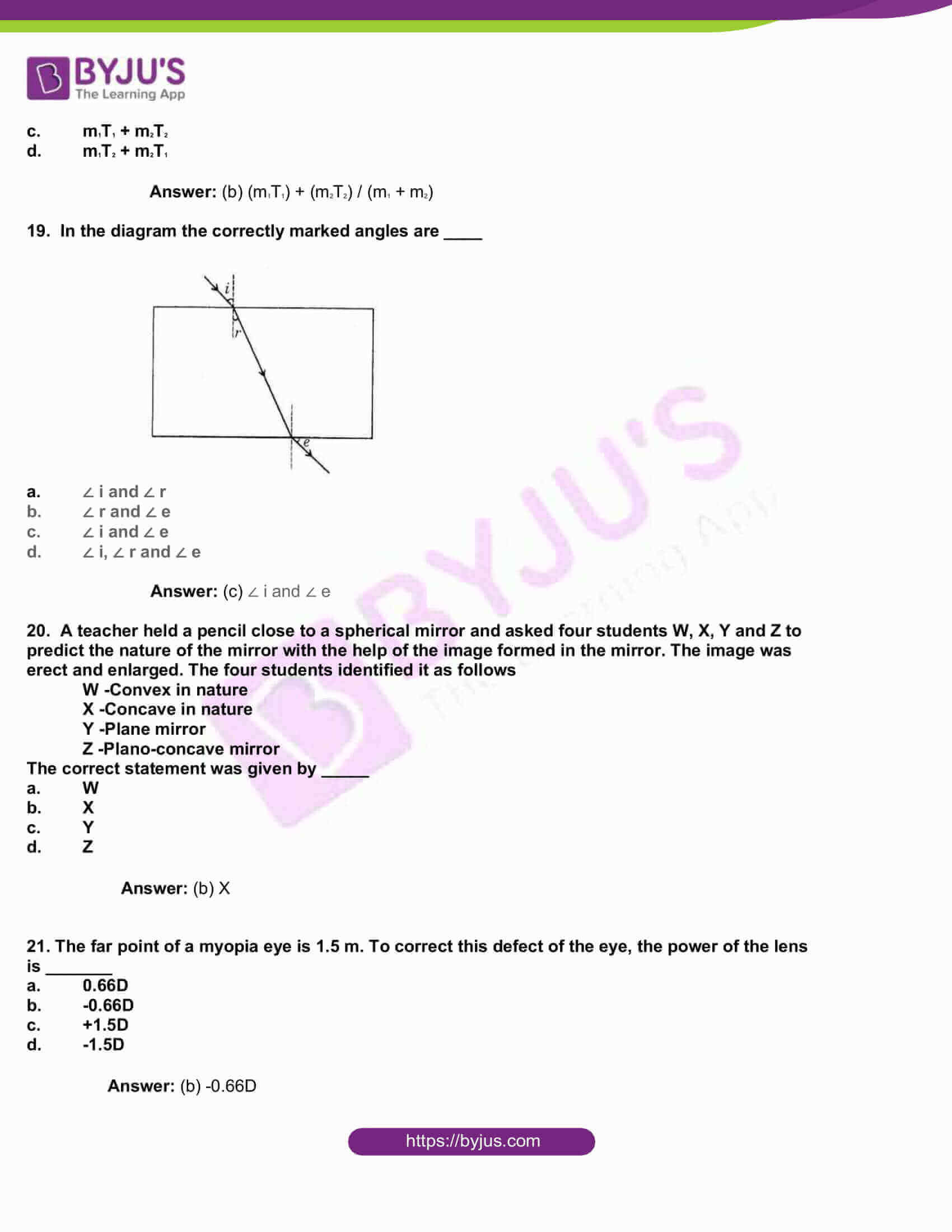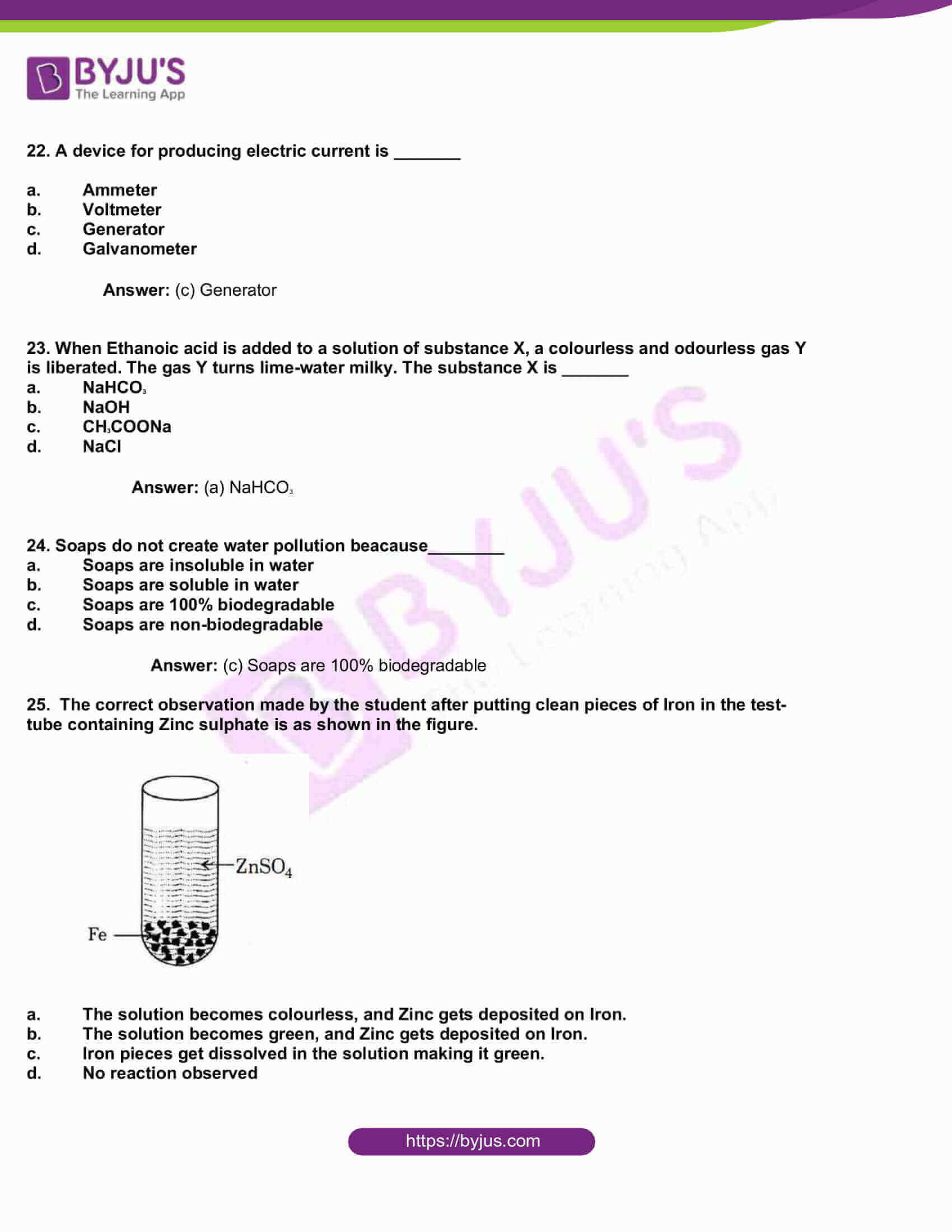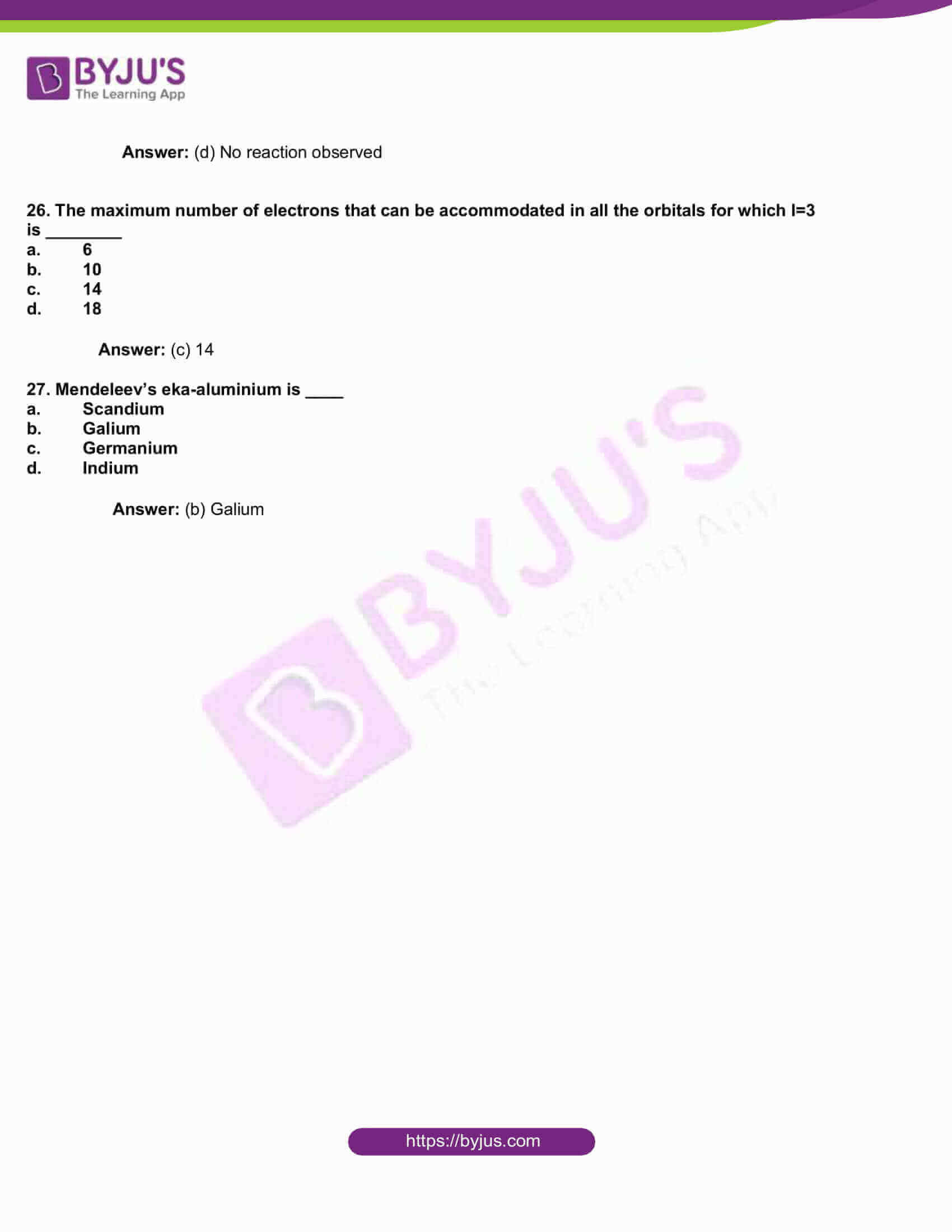Section-I 4 X 4 =16

1. An object is placed at the following distances from a convex lens of focal length 10 cm.

(a) 8 cm

(b)15 cm

(c) 20 cm

(d) 25 cm

Which position of the object will produce____

(i) a diminished, real and inverted image?

(ii) a magnified, real and inverted image?

(iii) a magnified, virtual and erect image?

(iv) an image of the same size as the object?

Answer: Here, the focal length of the concave mirror is given as 10 cm. The focus of the concave mirror is at some 10 cm distance from the mirror, and the centre of curvature, marked as C is at a distance of 2 X 10 = 20 cm from the mirror.

(i) Normally, a diminished real image is formed by a concave mirror, when the object is placed beyond C, which is given as 20 cm here. So, in this question, the only distance beyond C is 25 cm. Hence, the position for the object that depicts a diminished, real and inverted image is at 25 cm.

(ii) Now, a magnified, real image is typically formed for an object placed between F=10 cm and C= 20 cm. So, the position of the object that will produce a magnified, real and inverted image is 15 cm.

Or

How do you verify that resistance of a conductor of uniform cross-section area is proportional to the length of the conductor at a constant temperature?

Answer: Use two rods of different lengths, though their resistance and the cross-section area of the rods remain the same. Some copper wires kept in the same temperature will have the same resistivity.

Suppose l and l1 are lengths of the wires.

Then, the formula for resistance of first wire of “l” length is R = p l/A

R = k l ( since p and A are constants)

As R is multiplied by L, this proves the R changes the same as L.

Hence, length is directly proportional to the resistance.

Now, for resistance of the second wire of length “ l1” is

R1 = p l1/A

R1 = k l1

Now, R / R’ = l / l’

2. Explain the process of melting and latent heat of fusion.

Or

2. (a) State the Right-hand rule with a labelled diagram.

(b) A coil of insulated copper wire is connected to a Galvanometer. What happens, if a bar magnet is ______

(i) pushed into the coil?

(ii) withdrawn from inside the coil?

(iii) held stationary inside the coil?

Answer: (a) Take a wire which is carrying current in the right hand, then the thumb points the current direction, and all other fingers surrounding the wire give the magnetic field direction due to the current. The right-hand rule is useful to find the magnetic force as it becomes easy to visualise the direction as provided in Lorentz force law.

For the right-hand rule and image check here.

(b) (i) The galvanometer will deflect if it is pushed into the coil

(ii) The galvanometer will deflect when the magnet moves and then will be constant after a second as the magnet is withdrawn from inside the coil

(iii) The point of the galvanometer will rise and then stop at the same position as the magnet is held stationary inside the coil.

3. (a) Equal lengths of Magnesium ribbons are taken in two test-tubes X and Y. Hydrochloric acid is added to test-tube X and Acetic acid is added to test-tube Y. In which test-tube, the reaction will be more vigorous? Why?

(b) Name the four chemicals that are obtained from common salt and write their molecular formula.

Answer: (a) Here, the test-tube X is more likely to fizz more vigorously, as Magnesium ribbons and hydrochloric acid reacts to produce MgCl2 and H₂. Also, HCL is a more active acid while acetic acid is really weak. And it is seen that strong acid like HCL will dissociate completely to form H+ ions.

(b) Calcium Oxychloride- CaOCl2 , Ammonium Chloride- NH4Cl. Sodium Hydroxide-NaOH and Sodium Chloride-NaCl are the four chemicals obtained from common salt.

Or

3. (a) N2 (g) + O2 (g)+ Heat → 2NO (g)

What information do you get from the above equation? Comment.

(b) Write an activity about how you conduct an experiment to show that more reactive metals replace less reactive metals from their compounds.

Answer: (a) From this equation, we can conclude that 1 mole of Nitrogen combines with 1 mole of Oxygen in the presence of heat to give two moles of the new gas nitrogen monoxide.

(b) Add an iron nail to a copper sulphate solution. CuSO4 solution is blue. Since iron is more reactive than copper, it will displace copper to form FeSO4, thus changing the blue colour to green. The reaction is as given below:

Fe+CuSO4 →FeSO4 +Cu

4. Two elements X and Y belong to Groups 1 and 2, respectively, in the same period of the Periodic Table. Compare these elements to:

(i) some electrons in their outermost orbit.

(ii) their atomic size and their valencies.

(iii) their ionization energy and metallic character.

(iv) formulae of their chlorides and sulphates.

Answer: (i) Number of electrons in the outermost orbit of X and Y are 1 and 2 electrons, respectively. The number of outermost electrons is the same as the group number.

(ii) The atomic size decreases across the period. Hence, X is said to have greater atomic size.

(iii) The ionisation energy decreases along the period and metallic character increases, so that the ionisation energy of X is high, while the metallic character is low.

(iv) The formulae for Chlorides: XCl and YCl2 and Sulphates: X2SO4 and YSO4.

## ​

OR

4. The four metals A, B, C and D, are in turn added to the following solutions one by one. The observations made are tabulated below:

 Metal Iron(II) Sulphate Copper(II) Sulphate Zinc Sulphate Silver Nitrate A No reaction Displacement ____ _____ B Displacement ______ No reaction ______ C No reaction No reaction No reaction Displacement D No reaction No reaction No reaction No reaction

Answer the following based on the above information:

(i) Which is the most reactive metal? Why?

(ii) What would be observed. If “B” is added to a solution of Copper (II) Sulphate and why?

(iii) Arrange the metals, A, B, C, D in the order of increasing reactivity.

(iv) Which one among A, B, C and D metals can be used to make containers that can be used to store any of the above solutions safely?

Answer: (i) B is the most reactive metal, as it displaces iron from its Iron Sulphate

(ii) Since B is more reactive than copper, if added to Copper sulphate, it will displace Cu from CuSO4 solution.

(iii) D < C <A < B is the metals in the increasing order of reactivity

(iv) D is the less reactive metal and so cannot displace any metal from its salts. Be it Zn or Ag, none of these can be displaced by D. Hence, containers made of D can be used to store any of the above solutions.

Section-II 6 X 2 =12

5. Suggest the reasons for the phenomenon associated with the following:

(i) the sky appearing blue

(ii) the twinkling stars

Answer: (i) When sunlight passes through the atmosphere, the fine particles in the air scatter the blue colour (shorter wavelengths) more strongly than red. The scattered blue light enters our eyes. Within the visible range of light, red light waves are scattered the least by atmospheric gas molecules. So, at sunrise and sunset, when the sunlight travels a long path through the atmosphere to reach our eyes, the blue light has been mostly removed, leaving mostly red and yellow light remaining. Blue light is scattered more than the other colours because it travels as shorter, smaller waves. This is why we see a blue sky most of the time.

(ii) Stars appear to twinkle when they are seen from the surface of the earth. Learn from here on why stars appear to twinkle.

6. Draw the diagram that explains the formation of an image by a plane mirror.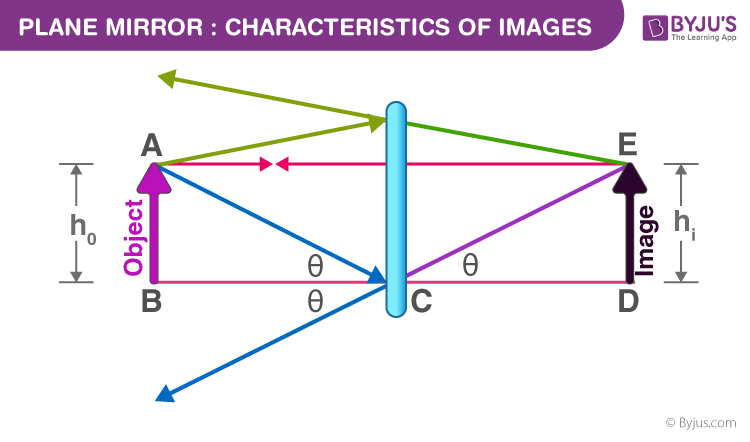7. A ray of light enters from air to a medium X. The speed of light in the medium is 1.5 x 108 m/s and the speed of light in air is 3 x 108 m/s. Find the Refractive index of the medium X.

Answer: Applying the snell’s law, the refractive index of the medium X will be

X = c / v, where c is the speed of light in air, while v is the speed of light in medium X.

Hence, Refractive index of X = (3 x 108) / (1.5 x 108) = 2.

8. For a better understanding of the electronic configuration in an atom, the teacher wrote a shorthand notation nlx on the blackboard. Looking at the notation, what could be the probable questions that generate in the student’s mind? Write any two of them.

Answer: Looking at the notation nlx, the probable questions that generate in the student’s mind are given below:

(i) What does n stand for?

(ii) What does this represent?

9. Represent the following molecules using Lewis notation:

1. Calcium and Chlorine to form Calcium chloride
2. Formation of Oxygen molecule from Oxygen atoms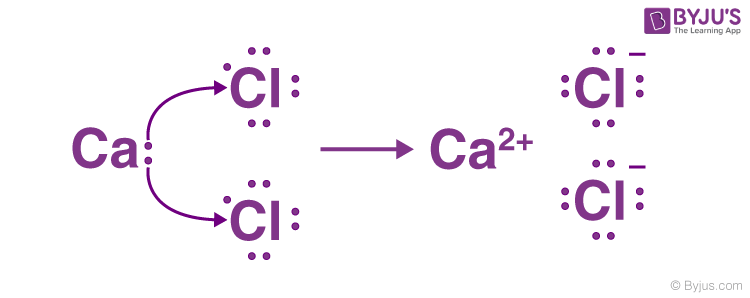(b)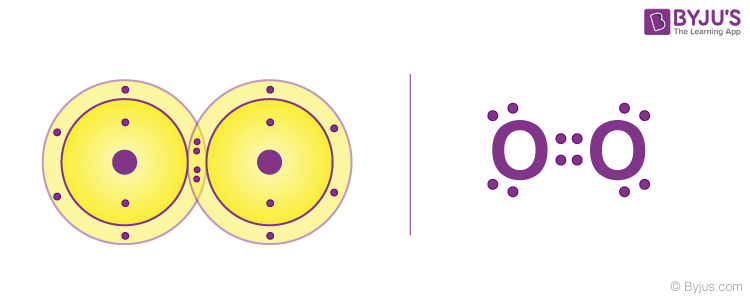10. (a) Why are vegetable oils healthy as compared to vegetable ghee?

(b) CH3-CH-CH=CH2 has the IUPAC name___________

|

Cl

Answer: (a) Oil is unsaturated and breaks down quickly, while ghee is saturated. Hence, the bonds in ghee cannot be easily broken. Saturated fatty acids are considered as harmful for our health. Thus, vegetable oils are healthy as compared to ghee.

(b) 2−chlorobut−3−ene is the IUPAC name. Here, chlorine is found in the second position.

Double bond is present, therefore ene. Meanwhile, the longest carbon chain is of 4 carbons with butane as the parent carbon.

Section-III 7 X 1 = 7

11. What is the cause of Presbyopia ?

Answer: Presbyopia can be confused with hyperopia as the symptoms present themselves in the same way. Close objects appear blurred for the person with presbyopia. This is caused as a part of the natural ageing process of the eye. The ciliary muscles lose their ideal functioning power over time and do not work effectively. Even the crystalline lens loses its flexibility over time (it starts hardening), thus affecting its focusing power. Even if someone has never had any vision problems in their life, they may still very well be subject to the effects of presbyopia. It occurs between the ages of 40 – 60.

12. Draw a ray diagram to show the angle of deviation when a ray of light passes through a glass prism.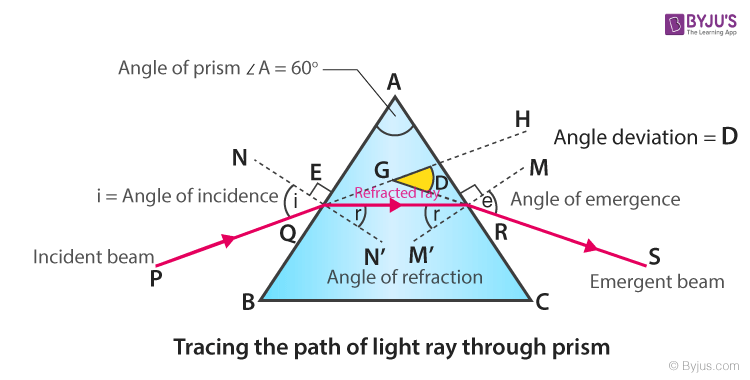13.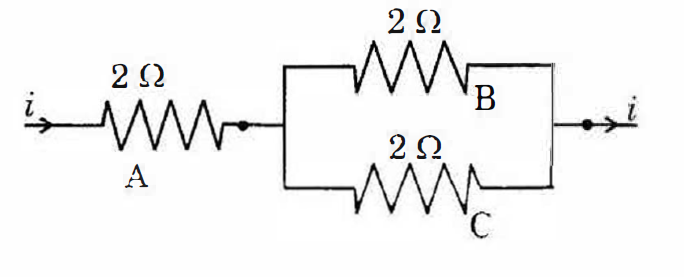Three resistors A, B and C are connected as shown in the figure. Each of them dissipates energy to a maximum of 18 W. Find the maximum current that can flow through the three resistors.

Answer: Given here are Voltage across the resistor V, I as current across the resistor and R is the value of resistance.

Power (P) dissipated by the resistor = V² / R

Hence, P = I² R

Each recipient dissipates energy = 18 W.

Maximum current via B or C or A is, I² = 18 Watts/2 ohms

So, I = 3 Ampere maximum

As the resistances B and C are connected in parallel, the current that comes from the resistance A is divided equally between the resistance B and C.

Therefore, current via B = current via resistance C = 1/2 * current through A

Hence, as the current passing through resistor A is max = 3 ampere, then the current through resistor B and resistor C are 1.5 Amperes maximum.

14. What happens when a current carrying wire is placed in a magnetic field?

Answer: When a current carrying wire is placed in a magnetic field, it experiences a magnetic force. Meanwhile, the direction of force is explained by Flemming’s Left-Hand Rule.

15. On adding dilute Hydrochloric acid to Copper oxide powder, the solution formed is blue-green. Write the new compound formed.

Answer: When dilute Hydrochloric acid is added to Copper oxide powder, a new compound Copper chloride is formed, and water is released in this reaction. This reaction is also known as displacement reaction.

16. Draw a neat labelled diagram of a Reverberatory furnace.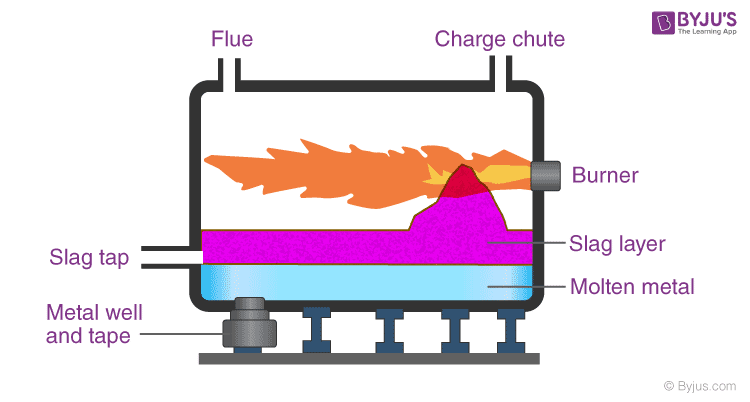17. How do you explain the role of Oxygen in the combustion process?

Answer: Oxygen plays a significant role in combustion. It functions as an oxidiser, thus supporting combustion.

Section-IV 10 X ½ = 5

18. The temperature (T) of two samples of the same substance with masses m1 and m2 and temperatures T1 and T2, when added together is_________

1. (m2T1) – (m1T2) / (m1-m2)
2. (m1T1) + (m2T2) / (m1 + m2)
3. m1T1 + m2T2
4. m1T2 + m2T1

Answer: (b) (m1T1) + (m2T2) / (m1 + m2)

19. In the diagram the correctly marked angles are ____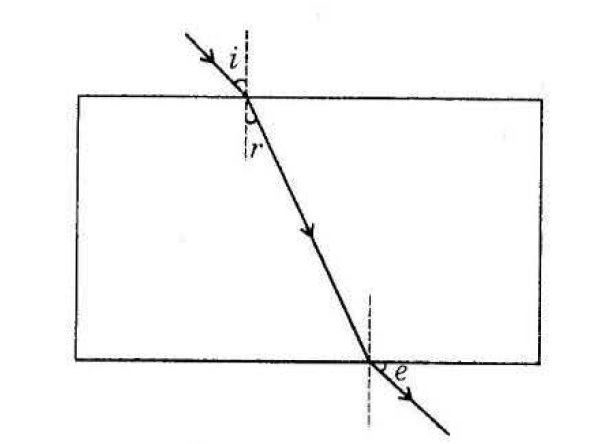1. ∠ i and ∠ r
2. ∠ r and ∠ e
3. ∠ i and ∠ e
4. ∠ i, ∠ r and ∠ e

Answer: (c) ∠ i and ∠ e

20. A teacher held a pencil close to a spherical mirror and asked four students W, X, Y and Z to predict the nature of the mirror with the help of the image formed in the mirror. The image was erect and enlarged. The four students identified it as follows

W -Convex in nature

X -Concave in nature

Y -Plane mirror

Z -Plano-concave mirror

The correct statement was given by _____

1. W
2. X
3. Y
4. Z

21. The far point of a myopia eye is 1.5 m. To correct this defect of the eye, the power of the lens is _______

1. 0.66D
2. -0.66D
3. +1.5D
4. -1.5D

22. A device for producing electric current is _______

1. Ammeter
2. Voltmeter
3. Generator
4. Galvanometer

23. When Ethanoic acid is added to a solution of substance X, a colourless and odourless gas Y is liberated. The gas Y turns lime-water milky. The substance X is _______

1. NaHCO3
2. NaOH
3. CH3COONa
4. NaCl

24. Soaps do not create water pollution beacause________

1. Soaps are insoluble in water
2. Soaps are soluble in water

25. The correct observation made by the student after putting clean pieces of Iron in the test-tube containing Zinc sulphate is as shown in the figure.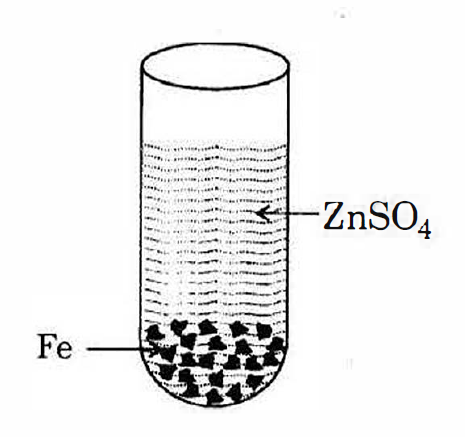1. The solution becomes colourless, and Zinc gets deposited on Iron.
2. The solution becomes green, and Zinc gets deposited on Iron.
3. Iron pieces get dissolved in the solution making it green.
4. No reaction observed

26. The maximum number of electrons that can be accommodated in all the orbitals for which l=3 is ________

1. 6
2. 10
3. 14
4. 18